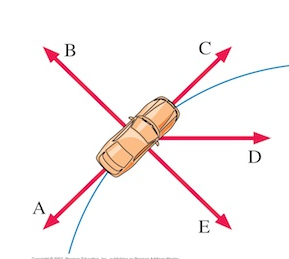# Problem: As seen from above, a car rounds a curved path shown below at a constant speed. Which of the vectors arrow shown best represents the net force acting on the car at the point shown?

###### FREE Expert Solution

The car is moving on a circular path with a constant speed.

Therefore, there will be no tangential force or tangential acceleration.

Only the centripetal force will be acting on the car.

90% (367 ratings)###### Problem Details

As seen from above, a car rounds a curved path shown below at a constant speed.

Which of the vectors arrow shown best represents the net force acting on the car at the point shown?